﻿ Stretched-exponential Decay of the Luminescence in Er<SUP>3+</SUP>-doped Te<SUB>2</SUB>-ZnO GlassesInternational Journal of Optics and Applications

p-ISSN: 2168-5053    e-ISSN: 2168-5061

2019;  8(1): 8-11

doi:10.5923/j.optics.20190801.02### Stretched-exponential Decay of the Luminescence in Er3+-doped Te2-ZnO Glasses

Abdelfatteh Cherif1, 2, Sami Youssef1

1Common First Year Deanship, UMM Al-Qura University, Saudi Arabia

2Institut Préparatoire aux Etudes d'Ingénieurs de Kairouan, Université de Kairouan, Tunisie

Correspondence to: Abdelfatteh Cherif, Common First Year Deanship, UMM Al-Qura University, Saudi Arabia.
 Email: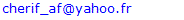Abstract

We present a time-resolved emission decay curves for 4S3/2à4I15/2 transition in Er3+- doped zinc tellurite glass, with the aim to interpret non-exponential decay. PL results can be described by the stretched exponential function. In this study, we address that this function enables us to a multi-configurational distribution of luminescent ions in a disordered medium.

Keywords: Energy Transfer, Decay Time, Erbium

Cite this paper: Abdelfatteh Cherif, Sami Youssef, Stretched-exponential Decay of the Luminescence in Er3+-doped Te2-ZnO Glasses, International Journal of Optics and Applications, Vol. 8 No. 1, 2019, pp. 8-11. doi: 10.5923/j.optics.20190801.02.

### 1. Introduction

The analysis of complex luminescence decays explained the dynamics of recombination center yields. The relaxation of different physical systems has been found to follow the stretched-exponential law. In particular, the photoluminescence from glassy materials and other solids [1-3] have been reported in the literature to behave this way.
In this paper we present the fluorescence decay profiles of 4S3/2à4I15/2 transition in Er3+- doped zinc tellurite glass (TZG) at various doping concentrations between 0.2 and 3% (molar). We show that the fluorescence decay profile can be fitted with the stretched exponential function. We have explained that lattice defect sites may interact through a dipolar energy transfer mechanism [4-7].

### 2. Experimental Details

Glasses were prepared from oxide powders of TeO2, ZnO and Er2O3 as starting materials using the conventional melt-quenching method. The material used in our measurement has a composition of 70TeO2–30ZnO. The amount of dopant was varied between 0.2 and 3 mol% Er2O3. The intrinsic lifetimes of the levels were obtained by exciting the samples with a laser analytical systems dye laser pumped by a pulsed frequency doubled Nd: YAG laser from BM Industries. The duration of pulses was 8 ns. The emitted light has been focused on a Jobin–Yvon HR S2 spectrophotometer. The detection has been performed using an R 1767 Hammamatsu photomultiplier and a Lecroy 9410 averager oscilloscope. All experiments were performed at room temperature.

### 3. Results and Discussion

The luminescence decay of homogeneously doped TeO2-ZnO: Er3+ was non-exponential due to non radiative energy transfer processes. Analysis of the photoluminescence decay profiles of Er3+ showed that the energy transfer between Er3+ ions was active only for concentrations beyond 0.5 mol%.
We have investigated the luminescence decay of the 4S3/2à4I15/2 transition in order to analyze the dynamics of this energy transfer. Figure 1 depicts the photoluminescence decay profiles of Er3+, measured for different ion concentrations. The luminescence decay was strongly non-exponential with ion concentration dependent time constant. We have found that the rapid decay of the relaxation curve increases with ion concentrations. It is shown that the experimental results of the photoluminescence decay can be described by the stretched exponential function: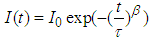(1)
Where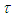is an effective time constant and β is a constant between 0 to 1.
For values β < 1 the decay is slower than the comparable exponential decay with the samefactor.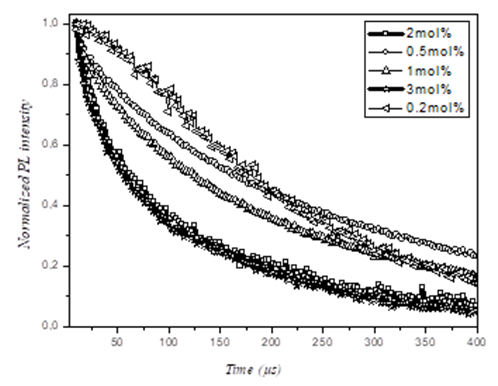Figure 1. Stretched exponential fit to the decay from the 4S3/2 level of the TZG, under 550 nm pumping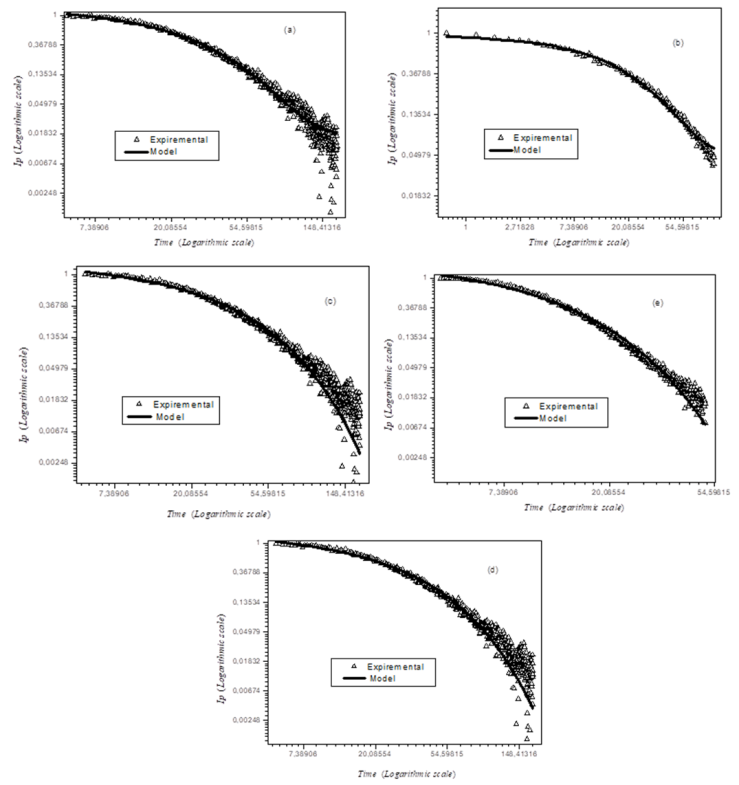Figure 2. The normalized intensity of the photoluminescence versus the normalized time, for 0.2 (a), 0.5(b), 1(c), 2(d) and 3(e) mol% Er3+ concentration
The values of measured parameters β and, obtained from the fit of photoluminescence decay data with use of equation 1 (in Figure 2). The parameters obtained from stretched exponential fits to the photoluminescence decay from the 4S3/2 level of the zinc tellurite glass samples are listed in Table 1, for different Er3+ ion concentrations ranging from 0.2 to 3 mol %. The function shows decreasing values of β with increasing concentration of Er3+. The stretched exponential spans the largest dynamic range of β (from 0.995 to 0.941). For the 0.2 mol % ion concentration, the stretched exponential function was very similar to the single exponential results with value of very close to one (0.995). This implies that there is one radiative recombination pathway for excited ions. This proposal is supported by the fact that energy transfer between excited ions can be ignored in the case of a low ion concentration . In the following, we assume that the dominant radiative and multiphonon decay times component reflect the total decay time, and obtainfrom the relation: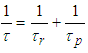(2)
where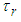is the radiative lifetime of the emitting level 4S3/2 and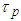is the multiphonon lifetime. To evaluate the first decay time, the intensity parameters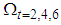have been calculated using the Judd-Ofelt formulae [9,10]. The obtained value for this lifetime is 203 µs with use of Ω2 = 1.15, Ω4 = 4.59 and Ω6 = 2.70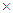10-20 cm2 respectively. The lifetimehas been computed using the model of multiphonon relaxation proposed by Riseberg and Moos  and for which this decay time is given by the relationship: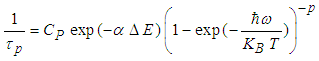(3)
where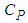and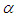are non-radiative parameters which depend on the host material,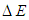represents the energy gap between two successive levels and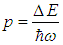is the number of phonons emitted in the relaxation process. In the case of the 4S3/2-4I15/2 transition, the value of, calculated from Eq. (3) at T=300 K, is of magnitude 1.55103 s. The parameters used for this calculation are: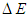= 7400 cm-1,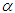= 4.710-3 cm,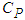= 6.31011 and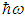≈ 750 cm-1 respectively . We will, now, study the effects of the Er3+ ion concentration on the total decay time. When this concentration increases, the energy transfer takes place. Thus the decay time characterizing this process must be considered in the calculation of. We labeled this decay time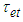. Then, Eq. (3) will be rewritten as: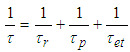(4)
Using the latter equation, we can deduce the energy transfer rate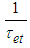from the photoluminescence intensity measurements.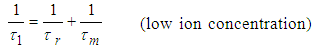(5)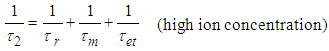(6)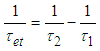(7)
Where τr, τm, τet are the radiative, multiphonon and energy transfer lifetimes of the 4S3/2 level, respectively.
Figure 3 displays the decay rate as a function of the square of the Er3+ ion concentration.
The linear behavior indicates that energy transfer process due to dipole-dipole interaction in Er3+- Er3+ pairs. The theory for this process was initially developed by Dexter for multi-polar coupling , the energy transfer rate occurring by an electric dipole interaction is proportional to the inverse of the sixth power of the distance separating the two ions and consequently to the squared concentration. This model supposes that the Er3+ ion pairs are homogeneously distributed in the host lattice.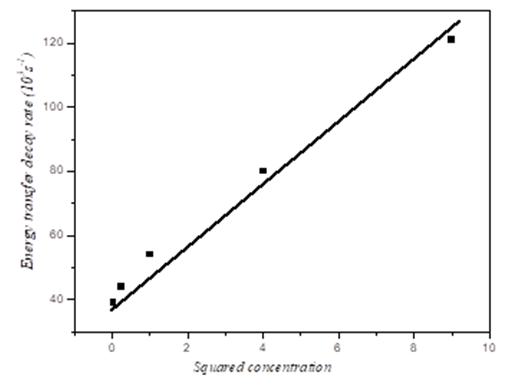Figure 3. The decay rate as a function of the square of the Er3+ ion concentration
If the observed non-exponential decay is a result of inter-ion energy transfer then, according to the expressions presented in Eq. 1, the photoluminescence decay should become increasingly non-exponential with donor concentration. On the other hand, if the non-exponential decay is a result of the finite distribution of multi-phonon decay rates caused by the inhomogeneous nature of the host glasses then the degree of non-exponentiality should be independent of donor concentration. But, the stretched exponential power obtained from the fits show a dependence on the Er3+ concentration, for concentrations as high as 0.5 mol%. This result found that the energy transfer process involving the 4S3/2 level has not a negligible effect on the decay dynamics of the 4S3/2 at these concentration levels. It can also be concluded from the presence of concentration dependence in this sample set, that inter-ion energy transfer mechanisms are the cause of the non-exponential behaviour from this manifold. Also, this leads to the second most probable cause for the reduction in the stretched exponential power, the host composition. The host composition has been shown a normal increase in fluorescence lifetime of the 4S3/2 level, which indicates that it has insignificant impact on the local environment surrounding the Er3+ ion. It is therefore proposed that the incorporation of Er3+ has not modified the local environment which could involve decreasing the amount of inhomogeneous broadening and therefore the low distribution of possible multi-phonon decay rates in the system.
 Table 1. Lifetimes and stretched exponential of the 4S3/2 level as a function of Er3+ concentration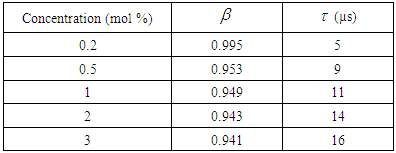### 4. Conclusions

A model of nonexponential behaviour is considered from the point of view of contribution of dipole-dipole interactions. We may conclude that the dipolar interaction dominates the energy transfer in this system and the incorporation of rare earth ions is made difficult by the structure of the network.
With this in mind, the reduction in stretched exponential power observed with the increase in the Er3+ concentration, may indicate that the energy transfer processes affect the decay dynamics of this level for Er3+ concentrations.

### References

  G. Schlegel, J. Bohnenberger, J. Potapova and A. Mews, Fluorescence decay time of single semiconductor nanocrystals., Physical Review Letters 88, 2002, pp.137401-137404.  R. Chen, Apparent stretched-exponential luminescence decay in crystalline solids., Journal of Luminescence 102-103, 2003, pp. 510-518.  B. R. Fisher, H. J. Eisler, N. E. Stott and M. G. Bawendi, Emission intensity dependence and single exponential behavior in single colloidal quantum dot fluorescence lifetimes., Journal of Physical Chemistry B 108, 2004, pp. 143-148.  D. L. Huber, Statical model for stretched exponential relaxation in macroscopic systems., Physical Review B 31, 1985, pp. 6070-6071.  F. Alvarez, A. Alegria and J. Colmenero, Relationship between the timedomain Kohlraush-Williams-Watts and frequency-domain Hayriliak-Negami relaxation functions., Physical Review B 44, 1991, pp. 7306-7312.  M. Lee, J. Kim, J. Tangand and R. M. Hochstrasser, Fluorescence quenching and lifetime distributions of single molecules on glass surfaces., Chemical Physics Letters 359, 2002, pp. 412-419.  I. Kuskovsky, G. F. Neumark, V. N. Bondarev and P. V. Pikhitsa, Decay dynamics in disorded systems: Application to heavily doped semiconductors., Physics Review Letters 80, 1998, pp. 2413-2416.  J. P. Van der Ziel, L. G. Van Uitert, W. H. Grodkiewicz and R. M. Mikulyak, 1.5 µm infrared excitation of visible luminescence in Y1-xErxF3 and Y1-x-yErxTmyF3 via resonant energy transfer., Journal of Applied Physics 60, 1986, pp. 4262-4267.  B. R. Judd, Optical Absorption Intensities of Rare-Earth ions., Physics. Review. 127, 750 (1962).  G. S. Ofelt, Intensities of Crystal Spectra of Rare-Earth ions., Journal of Chemical Physics, 137, 511 (1962).  L. A. Riseberg and H. W. Moos, Multiphonon Orbit-Lattice of Excited State of Rare-Earth ions in Crystals., Physics Review. 174, 429 (1968).  J. S. Wang, E. M. Vogel and E. Snitzer, Tellurite Glass: A New Candidate for Fiber Devices., Optical Materials 3, pp. 187-203 (1994).  D. L. Dexter, Cooperative optical Absorption in Solids., Physical Review 126, 1962, pp. 1962-1967.